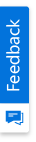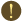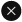We use cookies to give you the best experience on our website. If you continue to browse, then you agree to our privacy policy and cookie policy.Unfortunately, activation email could not send to your email. Please try again.# Helper class to support percentage sizing in GridControl/GridDataBoundGrid

Platform: WinForms |
Control: GridDataBoundGrid(Classic) |
Published Date: April 30, 2011 |
Last Revised Date: July 10, 2014

 The sample below has a helper class which, supports the automatic sizing in both a GridControl or GridDataBoundGrid in two ways. One way, which, is referred to as proportional sizing, is where all columns in the grid are equally sized. The other technique which, is referred to as percentage sizing, will allow you to specifiy that certain columns should occupy certain percentages of the available space for these columns. For an example of percentage sizing, assume that you have 5 columns in a grid. You can specify that column 5 must occupy 40% of the available space, and columns 1 and 2 must occupy 25% each of the available space. In this case, the helper class will take sum of the widths of the columns 1, 2, and 5. Widths are calculated based on percentage mentioned with the modified clientwidth. ie. Column 0 is kept undisturbed. It will then subtract this sum from the clientwidth. This will give the total space that is available for columns 3 and 4 which, would be 5% for each. In this manner, the columns will exactly occupy the client width of the grid. WireGrid and UnwireGrid are methods that are used to enable the sizing helper class. Proportional sizing is achieved using the QueryColWidth event. Percentage sizing will explicilty set the ColWidth[index] property for each column. C# public class GridColSizingHelper { private GridControlBase grid; public GridColSizingHelper(){} public bool WireGrid(GridControlBase grid, Hashtable ht) { int temp = 0; this.colPWidths = ht; for(int i = 1; i <= grid.Model.ColCount; ++i) { if(colPWidths.ContainsKey(i)) temp += int.Parse(colPWidths[i].ToString()); } // temp > 100 - unmodified column count if( temp > 100-(grid.Model.ColCount - colPWidths.Count)) return false; if(this.grid != null) UnwireGrid(); this.grid = grid; TurnOffProportion(); this.grid.ResizingColumns += new GridResizingColumnsEventHandler(grid_ResizingColumns); this.TurnOnPercentages(); return true; } bool WireGridFlag; public void WireGrid(GridControlBase grid) { if(this.grid != null) UnwireGrid(); this.grid = grid; grid.Model.Options.SmoothControlResize = false; this.grid.Model.QueryColWidth += new GridRowColSizeEventHandler(Model_QueryColWidth); this.grid.ResizingColumns += new GridResizingColumnsEventHandler(grid_ResizingColumns); grid.Refresh(); WireGridFlag = true; return; } public void UnwireGrid() { if(this.grid != null) { this.grid.ResizingColumns -= new GridResizingColumnsEventHandler(grid_ResizingColumns); this.grid.Model.QueryColWidth -= new GridRowColSizeEventHandler(Model_QueryColWidth); this.grid.Refresh(); this.reset(); this.grid = null; } } int clientsize, remainingSize; private void Model_QueryColWidth(object sender, GridRowColSizeEventArgs e) { if(e.Index > grid.Model.Cols.HeaderCount) { //clientsize = ClientSize - Row Headers this.clientsize = grid.ClientSize.Width - grid.Model.ColWidths.GetTotal(0, this.grid.Model.Cols.HeaderCount); e.Size = (int)clientsize / grid.Model.ColCount; e.Handled = true; } } public void TurnOffProportion() { if(WireGridFlag) { this.grid.Model.QueryColWidth -= new GridRowColSizeEventHandler(Model_QueryColWidth); WireGridFlag = false; } } } public void TurnOnPercentages() { try { int totalPercentSize = 0; this.clientsize = grid.ClientSize.Width - grid.Model.ColWidths.GetTotal(0, this.grid.Model.Cols.HeaderCount); for(int i = 1; i <= grid.Model.ColCount; ++i) { if(colPWidths.ContainsKey(i)) totalPercentSize += (int)clientsize * int.Parse(colPWidths[i].ToString())/100; } remainingSize =(int)(clientsize - totalPercentSize)/(grid.Model.ColCount - colPWidths.Count); // Setting the column widths from Hashtable passed through WireGrid for(int i = 1; i <= grid.Model.ColCount; ++i) { if(colPWidths.ContainsKey(i)) grid.Model.ColWidths[i] = (int)clientsize * int.Parse(colPWidths[i].ToString())/100; else grid.Model.ColWidths[i] = remainingSize; } } catch { MessageBox.Show("Make sure the Grid is wired before calling TurnOnPercentages()"); } } } private GridColSizingHelper Helper1; private GridColSizingHelper Helper2; private void Form1_Load(object sender, System.EventArgs e) { // There is a condition check in accepting hashtable values // ie.. the total of values passed must not be greater than (100 - Unmodified Column count) ht = new Hashtable(); ht.Add(1,20); ht.Add(9,30); ht.Add(4,13); // Attempts like using unwanted keys are not encouraged because count is being used //ht.Add(12,100); Helper1 = new GridColSizingHelper(); Helper2 = new GridColSizingHelper(); Helper2.WireGrid(this.gridControl1,ht); this.gridDataBoundGrid1.AllowResizeToFit = false; Helper1.WireGrid(this.gridDataBoundGrid1,ht); } VB Public Class GridColSizingHelper Private grid As GridControlBase Public Sub New() End Sub Public Function WireGrid(ByVal grid As GridControlBase, ByVal ht As Hashtable) As Boolean Dim temp As Integer = 0 Me.colPWidths = ht For i As Integer = 1 To grid.Model.ColCount If colPWidths.ContainsKey(i) Then temp += Integer.Parse(colPWidths(i).ToString()) End If Next i ' temp > 100 - unmodified column count If temp > 100-(grid.Model.ColCount - colPWidths.Count) Then Return False End If If Not Me.grid Is Nothing Then UnwireGrid() End If Me.grid = grid TurnOffProportion() AddHandler grid.ResizingColumns, AddressOf grid_ResizingColumns Me.TurnOnPercentages() Return True End Function Private WireGridFlag As Boolean Public Sub WireGrid(ByVal grid As GridControlBase) If Not Me.grid Is Nothing Then UnwireGrid() End If Me.grid = grid grid.Model.Options.SmoothControlResize = False AddHandler grid.Model.QueryColWidth, AddressOf Model_QueryColWidth AddHandler grid.ResizingColumns, AddressOf grid_ResizingColumns grid.Refresh() WireGridFlag = True Return End Sub Public Sub UnwireGrid() If Not Me.grid Is Nothing Then RemoveHandler grid.ResizingColumns, AddressOf grid_ResizingColumns RemoveHandler grid.Model.QueryColWidth, AddressOf Model_QueryColWidth Me.grid.Refresh() Me.reset() Me.grid = Nothing End If End Sub Private clientsize, remainingSize As Integer Private Sub Model_QueryColWidth(ByVal sender As Object, ByVal e As GridRowColSizeEventArgs) If e.Index > grid.Model.Cols.HeaderCount Then 'clientsize = ClientSize - Row Headers Me.clientsize = grid.ClientSize.Width - grid.Model.ColWidths.GetTotal(0, Me.grid.Model.Cols.HeaderCount) e.Size = CInt(Fix(clientsize)) / grid.Model.ColCount e.Handled = True End If End Sub Public Sub TurnOffProportion() If WireGridFlag Then RemoveHandler grid.Model.QueryColWidth, AddressOf Model_QueryColWidth WireGridFlag = False End If End Sub Public Sub TurnOnPercentages() Try Dim totalPercentSize As Integer = 0 Me.clientsize = grid.ClientSize.Width - grid.Model.ColWidths.GetTotal(0, Me.grid.Model.Cols.HeaderCount) For i As Integer = 1 To grid.Model.ColCount If colPWidths.ContainsKey(i) Then totalPercentSize += CInt(Fix(clientsize)) * Integer.Parse(colPWidths(i).ToString())/100 End If Next i remainingSize =CInt(Fix(clientsize - totalPercentSize))/(grid.Model.ColCount - colPWidths.Count) ' Setting the column widths from Hashtable passed through WireGrid For i As Integer = 1 To grid.Model.ColCount If colPWidths.ContainsKey(i) Then grid.Model.ColWidths(i) = CInt(Fix(clientsize)) * Integer.Parse(colPWidths(i).ToString())/100 Else grid.Model.ColWidths(i) = remainingSize End If Next i Catch MessageBox.Show("Make sure the Grid is wired before calling TurnOnPercentages()") End Try End Sub End Class Private Helper1 As GridColSizingHelper Private Helper2 As GridColSizingHelper Private Sub Form1_Load(ByVal sender As Object, ByVal e As System.EventArgs) ' There is a condition check in accepting hashtable values ' ie.. the total of values passed must not be greater than (100 - Unmodified Column count) ht = New Hashtable() ht.Add(1,20) ht.Add(9,30) ht.Add(4,13) ' Attempts like using unwanted keys are not encouraged because count is being used 'ht.Add(12,100); Helper1 = New GridColSizingHelper() Helper2 = New GridColSizingHelper() Helper2.WireGrid(Me.gridControl1,ht) Me.gridDataBoundGrid1.AllowResizeToFit = False Helper1.WireGrid(Me.gridDataBoundGrid1,ht) End Sub Here is a sample that illustrates this: http://help.syncfusion.com/support/samples/kb/Grid.Windows/GridColumnWidthSizing/GridColumnWidthSizing.zip

2X faster development

The ultimate WinForms UI toolkit to boost your development speed.
ADD COMMENT
You must log in to leave a comment

#### Please sign in to access our KB

This page will automatically be redirected to the sign-in page in 10 seconds.You are using an outdated version of Internet Explorer that may not display all features of this and other websites. Upgrade to Internet Explorer 8 or newer for a better experience.